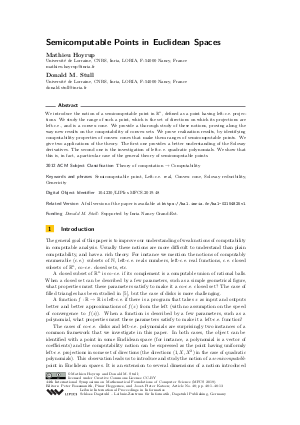Document# Semicomputable Points in Euclidean Spaces

### Authors Mathieu Hoyrup, Donald M. Stull## File

LIPIcs.MFCS.2019.48.pdf
• Filesize: 469 kB
• 13 pages

## Cite As

Mathieu Hoyrup and Donald M. Stull. Semicomputable Points in Euclidean Spaces. In 44th International Symposium on Mathematical Foundations of Computer Science (MFCS 2019). Leibniz International Proceedings in Informatics (LIPIcs), Volume 138, pp. 48:1-48:13, Schloss Dagstuhl - Leibniz-Zentrum für Informatik (2019)
https://doi.org/10.4230/LIPIcs.MFCS.2019.48

## Abstract

We introduce the notion of a semicomputable point in R^n, defined as a point having left-c.e. projections. We study the range of such a point, which is the set of directions on which its projections are left-c.e., and is a convex cone. We provide a thorough study of these notions, proving along the way new results on the computability of convex sets. We prove realization results, by identifying computability properties of convex cones that make them ranges of semicomputable points. We give two applications of the theory. The first one provides a better understanding of the Solovay derivatives. The second one is the investigation of left-c.e. quadratic polynomials. We show that this is, in fact, a particular case of the general theory of semicomputable points.

## Subject Classification

##### ACM Subject Classification
• Theory of computation → Computability
##### Keywords
• Semicomputable point
• Left-c.e. real
• Convex cone
• Solovay reducibility
• Genericity

## Metrics

• Access Statistics
• Total Accesses (updated on a weekly basis)
0